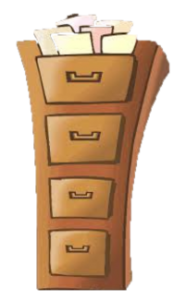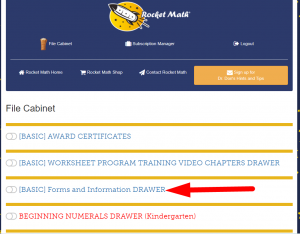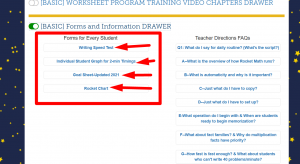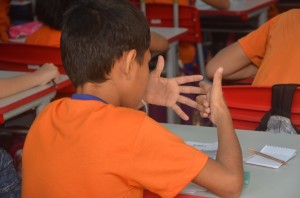# How to Homeschool or tutor with Worksheet Program

##Tutoring and Homeschooling

The Rocket Math Worksheet Program runs out of our virtual filing cabinet on the web. You get access by subscribing here and you can start with a \$20 trial for 60 days.

## Before you teach, you might assess with our Pre-Tests

If you don’t know what skills your student needs to learn, go to our Pre-Tests page here.  There are pre-tests for math facts, writing speed and for learning computation (multi-step arithmetic problems).

## (1) If help is needed with Math Facts

When you know your student needs help with math facts, view the video below that explains how you tutor with the Worksheet Program for the basic facts.

However, you might want to consider the Rocket Math Online Game which is less work for you. You can try it for 30-days for free.  It costs only \$9 a year per student seat. For math facts you can use that instead of the Worksheet Program.  In the Worksheet Program you’re going to be doing the tutoring.  In the Online Game the computer will be doing that work. Up to you!

You start printing forms you can find in the Forms and Information drawer in the Rocket Math virtual filing cabinet.Here you see the first forms you have to print out for your student.But first watch the video below to see how to do the standard Rocket Math facts practice program.

Besides the basic math facts for addition, subtraction, multiplication and division, the Rocket Math virtual filing cabinet has many other “Learning Tracks” in drawers that can be used by those who are home schooling or tutoring.

Mostly peer practice. Most of the Learning Tracks, (most of the drawers) follow this same pattern of how students practice with their checker or tutor for two minutes and then take a one-minute test to see if they are ready to move on.

### Here are two drawers or Learning Tracks that are teaching (rather than practice), for students just starting math instruction.

Beginning Numerals is for teaching children to count objects and match them with the correct numeral.  This is tutor-led instruction, right after students can count from memory to ten.  In the drawer for this program is a video with suggestions on how to teach this.

Conceptual Addition is for teaching children the concept of addition. For the first four worksheets you and the child count the objects in both groups to see how many total are there.  Then starting on set E you teach how to “count on” from a number other than one.  So you get five going and count the other three objects as six, seven, eight.  Showing that 5 plus 3 equals 8.  In the drawer there are teaching script and suggestions and a video.

## (2) If help is needed for writing numerals quickly

If you gave the writing speed test before testing math facts, and if your student completed 18 or fewer boxes in one minute, you might have noticed the recommendation for Rocket Writing for Numerals.  You may also give this pre-test for the Rocket Writing for Numerals program.  If a student cannot write 40 digits in one minute on the pre-test, then they need to go through this program.

Rocket Writing for Numerals is for teaching children how to form the numerals 0 through 9 correctly, efficiently, and legibly.  There are 72 pages of practice divided into four chapters (each 18 worksheets long) which gradually increase in difficulty. In the first chapter students learn how to form them by tracing them.  In the second chapter they trace and then write numerals without tracing. In the third chapter they learn to write them without tracing and efficiently enough to take a timing.  In the fourth chapter they get up to the speed, 40 digits per minute, needed for Rocket Math.

## (3) If help is needed for learning computation

###But only if your student is ready.

If students  have finished a math facts learning track (add, subtract, multiply or divide), then they can go forward and learn computation in that operation.  Computation is how to do multi-step arithmetic problems.  It is very important that students have learned the facts first!  The meanest thing a teacher can do is make students do computation before they’ve memorized the facts!  It is just too hard if you have to figure out or worse yet, count on your fingers.  Don’t do that.  Finish the Rocket Math facts in the basic operations, before starting on computation in that operation.

There are four learning tracks in computation.  They are organized in a similar fashion.  The video below gives an overview of how to use these materials to help your student learn arithmetic.

Addition–Learning Computation is a program for teaching all the necessary skills for doing addition computation.  You MUST get your student through Addition facts first before starting in on computation, please!! There is a placement test you can use to find the skills where they need to begin instruction.  There is a video that explains how the program works and how to teach it.

Subtraction–Learning Computation is a program for teaching all the necessary skills for doing subtraction computation.  You MUST get your student through Subtraction facts first before starting in on computation, please!! There is a placement test you can use to find the skills where they need to begin instruction.  There is a video that explains how the program works and how to teach it.

Multiplication–Learning Computation is a program for teaching all the necessary skills for doing multiplication computation.  You MUST get your student through Multiplication facts first before starting in on computation, please!! There is a placement test you can use to find the skills where they need to begin instruction.  There is a video that explains how the program works and how to teach it.

Division –Learning Computation is a program for teaching all the necessary skills for doing division computation.  You MUST get your student through Division facts first before starting in on computation, please!! There is a placement test you can use to find the skills where they need to begin instruction.  There is a video that explains how the program works and how to teach it.

##Set up a Zoom meeting with Dr. Don.

His favorite thing to talk about is instruction, so he’s happy to help.